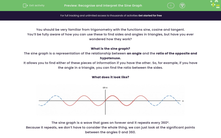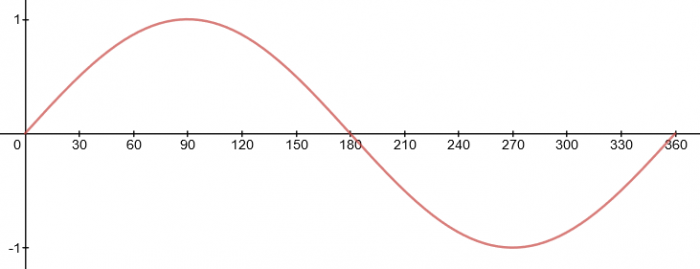# Recognise and Interpret the Sine Graph

In this worksheet, students will look at the sine graph, its significant points and how to use it to find alternative values.Key stage:  KS 4

Year:  GCSE

GCSE Subjects:   Maths

GCSE Boards:   AQA, Eduqas, OCR, Pearson Edexcel,

Curriculum topic:   Algebra, Graphs of Equations and Functions

Curriculum subtopic:   Graphs Graphs of Equations and Functions

Difficulty level:#### Worksheet Overview

You should be very familiar from trigonometry with the functions sine, cosine and tangent.

You’ll be fully aware of how you can use these to find sides and angles in triangles, but have you ever wondered how they work?

What is the sine graph?

The sine graph is a representation of the relationship between an angle and the ratio of the opposite and hypotenuse.

It allows you to find either of these pieces of information if you have the other. So, for example, if you have the angle in a triangle, you can find the ratio between the sides.

What does it look like?The sine graph is a wave that goes on forever and it repeats every 360°.

Because it repeats, we don’t have to consider the whole thing, we can just look at the significant points between the angles 0 and 360.

What are the significant points?From this, we can see that there are some significant points we need to consider:

The maximum: The highest value that sin (x) can have is 1. This occurs at 90°.

The minimum: The lowest value that sin (x) can have is -1. This occurs at 270°.

Intercepts: The function sin (x) crossed the x axis at 0°, 180° and 360°.The two angle problem

Getting one is easy, you can just use the sin-1 function on your calculator. The problem is how do you find the other?

This is all about understanding the sine graph.

Example:

There are two angles with sin (x) = 0.5, x1 = 30°. Find x2

To solve this, we need to look at the sine graph:We can see here that the second angle is 150°. The reason for this is that the sine graph is symmetrical between 0 and 180 (and between 180 and 360).

Usefully, there is a nice little rule:

For a sine graph:  x2 = 180 – x1

### What is EdPlace?

We're your National Curriculum aligned online education content provider helping each child succeed in English, maths and science from year 1 to GCSE. With an EdPlace account you’ll be able to track and measure progress, helping each child achieve their best. We build confidence and attainment by personalising each child’s learning at a level that suits them.

Get started